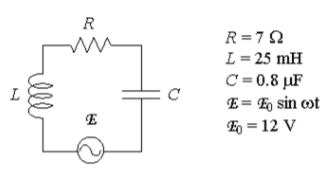# Problem: A series RLC circuit with L = 25 mH, C = 0.8 μF and R = 7Ω is driven by a generator with a maximum emf of 12V and a variable angular frequency ω. If the inductance L is doubled (keeping all other quantities given, including the generator frequency, the same), compared to the power dissipated when the circuit was at resonance, the power now dissipated in the resistor willa) increase.b) decrease.c) stay the same.

###### Problem Details

A series RLC circuit with L = 25 mH, C = 0.8 μF and R = 7Ω is driven by a generator with a maximum emf of 12V and a variable angular frequency ω. If the inductance L is doubled (keeping all other quantities given, including the generator frequency, the same), compared to the power dissipated when the circuit was at resonance, the power now dissipated in the resistor will

a) increase.

b) decrease.

c) stay the same.